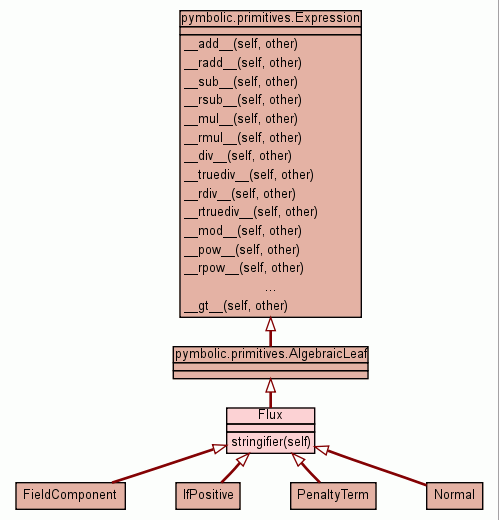[frames] | no frames]

# Class Flux

source codeInstance Methods

 stringifier(self) source code

Inherited from `pymbolic.primitives.Expression`: `__add__`, `__call__`, `__div__`, `__eq__`, `__float__`, `__ge__`, `__getitem__`, `__gt__`, `__hash__`, `__le__`, `__lt__`, `__mod__`, `__mul__`, `__ne__`, `__neg__`, `__pow__`, `__radd__`, `__rdiv__`, `__repr__`, `__rmul__`, `__rpow__`, `__rsub__`, `__rtruediv__`, `__str__`, `__sub__`, `__truediv__`, `get_hash`, `is_equal`

Inherited from `object`: `__delattr__`, `__getattribute__`, `__init__`, `__new__`, `__reduce__`, `__reduce_ex__`, `__setattr__`

 Properties

Inherited from `object`: `__class__`

 Method Details

### stringifier(self)

source code
Overrides: pymbolic.primitives.Expression.stringifier

 Generated by Epydoc 3.0.1 on Sat Aug 29 14:33:27 2009 http://epydoc.sourceforge.net